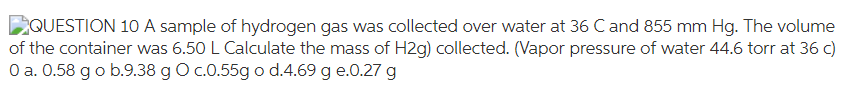# Problem: A sample of hydrogen gas was collected over water at 36°C and 855 mm Hg. The volume of the container was 6.50 L. Calculate the mass of H2 (g) collected. (Vapor pressure of water = 44.6 torr at 36°C) a. 0.58 g b. 9.38 g c. 0.55 g d. 4.69 g e. 0.27 g

###### FREE Expert Solution
80% (376 ratings)###### Problem Details

A sample of hydrogen gas was collected over water at 36°C and 855 mm Hg. The volume of the container was 6.50 L. Calculate the mass of H2 (g) collected. (Vapor pressure of water = 44.6 torr at 36°C)

a. 0.58 g

b. 9.38 g

c. 0.55 g

d. 4.69 g

e. 0.27 g